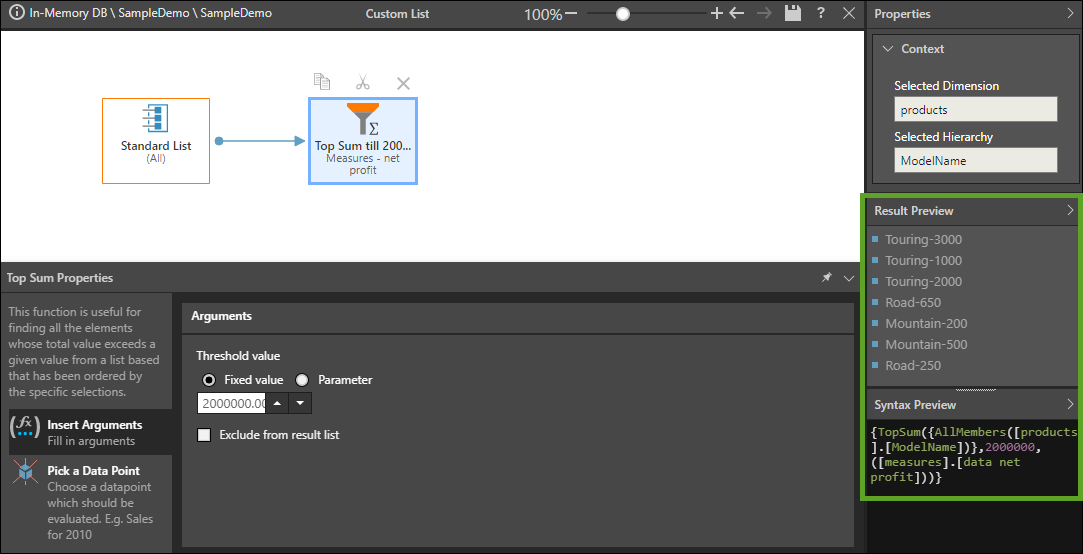# Top Sum

Returns a list of top member elements from derived from the given list, that sum to a specified value. The logic for deriving the list will use the optional numeric data point (which can be a fixed value or a parameter) if supplied. Otherwise, it uses the default selections for the model or the context in which the function was used.

#### Syntax & Usage

TopSum([LIST], counter, optional numeric data-point)
• Create a list from which you want to derive the top sum list.
• Add the Top Sum block to the list, and set the counter from the Insert Arguments window under "Number of Items". This can be a static number or a numeric parameter.
• Select "Exclude from Result Set" if you want to remove the top sum elements from the list (as opposed to creating a list of top sum elements).

#### Example

This expression returns a list of the top Products by Net Profit that sum to 2,000,000; the given list is the Products hierarchy, and the Top Sum block is assigned a set value of 2,000,000 percent and Net Profit as the data point:

{TopSum({AllMembers([products].[ModelName])},2000000,([measures].[data net profit]))}